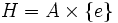# Normal not implies potentially verbal

This article gives the statement and possibly, proof, of a non-implication relation between two subgroup properties. That is, it states that every subgroup satisfying the first subgroup property (i.e., normal subgroup) need not satisfy the second subgroup property (i.e., potentially verbal subgroup)
View a complete list of subgroup property non-implications | View a complete list of subgroup property implications
Get more facts about normal subgroup|Get more facts about potentially verbal subgroup
EXPLORE EXAMPLES YOURSELF: View examples of subgroups satisfying property normal subgroup but not potentially verbal subgroup|View examples of subgroups satisfying property normal subgroup and potentially verbal subgroup

## Definition

It is possible to have a group$G$ and a normal subgroup$H$ of$G$ that is not a potentially verbal subgroup, i.e., there is no group$K$ containing$G$ for which$H$ is a verbal subgroup of$K$.

## Proof

### Example involving complete group

Let$A$ be a nontrivial complete group. Define$G := A \times A$ and$H := A \times \{ e \}$. Clearly,$H$ is a normal subgroup of$G$.

Suppose$K$ is a group containing$G$, such that$H$ is verbal in$K$. In particular,$H$ is normal in$K$. Since$H$ is complete, it is a direct factor, so there exists a group$C$ that is a complement to$H$, so$K = H \times C$ as an internal direct product. Further, since$G/H \cong H \cong A$ is a subgroup of$K/H$,$C$ has a subgroup, say$B$, isomorphic to$A \cong H$.

Then, consider the endomorphism$\alpha$ of$K$ that sends$C$ to the trivial subgroup and$H$ isomorphically to the subgroup$B$ does not send$H$ to within itself. Thus,$H$ is not fully invariant in$K$, and hence,$H$ is not verbal in$K$.

### Example involving infinite simple non-abelian group

Suppose$A$ is an infinite simple non-abelian group that satisfies no nontrivial identity. An example is the finitary alternating group on an infinite set (see finitary alternating group on infinite set implies no nontrivial identity, alternating groups are simple). Define$G = A \times A$ and$H = A \times \{ e \}$ is the first direct factor of$G$. Let$H_2 = \{ e \} \times H$ be the second direct factor.

•$H$ is a direct factor of$G$: This is direct from the definition.
•$H$ is not a potentially verbal subgroup of$G$:
1. For any nontrivial word$w$, the verbal subgroup generated by that with letters from$H$ is the whole of$H$: the verbal subgroup corresponding to that word, with letters coming from$H$, is a verbal subgroup of$H$. Hence, it is normal in$H$, and so is either trivial or the whole group. It is not trivial, because$H$ satisfies no nontrivial identity, so it is the whole group.
2. For any nontrivial word$w$, the verbal subgroup generated by that with letters from$H_2$ is the whole of$H_2$: This follows for the same reason as the previous step.
3. For any nontrivial word$w$, the verbal subgroup generated by that with letters from$G$ is the whole of$G$: This follows from the previous step.
4. If$G$ is a subgroup of a group$K$, any nontrivial verbal subgroup of$K$ contains$G$. In particular, it cannot be equal to$H$: This is because any nontrivial word$w$ gives rise to a subgroup containing$G$, because even restricting the letters to within$G$, we obtain the whole of$G$.